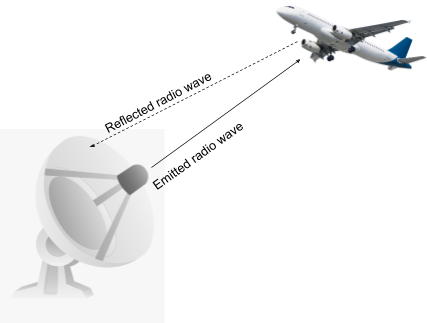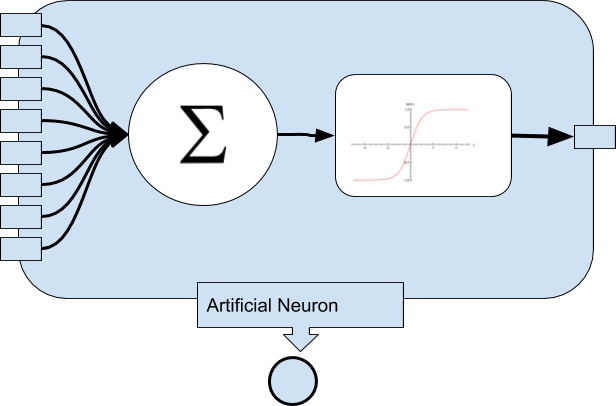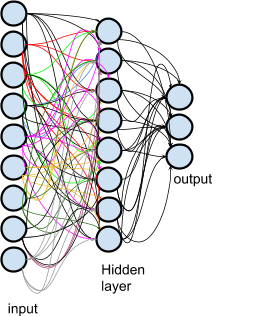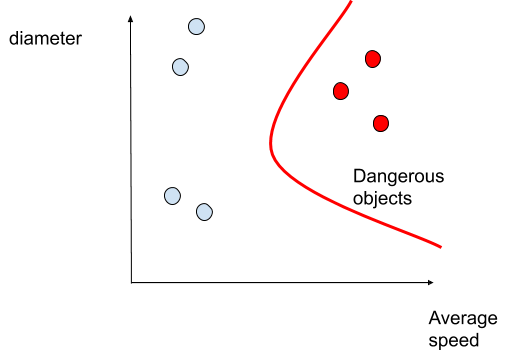•written on Dec 16, 2019
by Martin Rupp

### Artificial Intelligence: Important Aspects of Neural Networks Applied to Radar Systems

ATCO ATSEP Artificial Intelligence Theory & ResearchRADAR is short for RAdio Detection And Ranging. The principle of RADAR systems is not very difficult to understand. It is simply because most solid objects reflect radio waves, e.g., electromagnetic radiation with wavelengths superior to infrared. A RADAR therefore sends radio waves through an emitter and captures the “response”, e.g the reflecting signal through a receiver.

The whole system gives information about distant objects: their shapes, dimensions, locations and velocity - among other things.

RADARs are very usefull and increasingly applied in a growing variety of use cases. They are traditionally used in civil aviation and in the military, to detect aircraft or eventual threats from enemies (ships, planes, rockets etc…).# Neural networks

## History

Neural networks are non-symbolic machine learning systems, which use a very basic and primitive modelling of the human brain to provide classification of data. Neural networks use basic processing units - artificial neurons - organised in layers and a training mechanism. They have been developed as early as in the 60’s. The Perceptron machine (1958) was the first of the neural networks machines, developed for image recognition by the U.S Navy. Since then they have evolved to multi-layer architectures (deep learning).

## Artificial neurons

An artificial neuron is an abstract computer structure, which acts as a basic processing unit. It receives N input signals and transform them into a single signal using a weighted sum, then it uses an activation function to fire the output signal(s).If Φ is the activation function, than the output signal is Φ (Σi=0...N mi Xi
w
here
- the mi are the N weights and the bias;
- the Xi are the N inputs and the bias input.# Artificial neural networks

An artificial neural network is made up of layers of artificial neurons. Layer is a generic term which encompasses a set of artificial neurons considered as 'nodes' and that are operating at a specific depth inside a neural network.

Layers are divided into three categories:

• The input layer;
• The Hidden layers;
• The output layer.

The input layer receives the input data to process. So each node of this layer can be seen as the input variable of a function.

The hidden layers contains the processing itself. These layers apply weights and thresholds to the inputs to generate the relevant outputs.

Each hidden layer is connected to the other, which is next to it so that they form a processing chain.

The output layer is at the end, this is the last layer and outputs the result.
The design of the layers is fundamental. The data is usually transformed, processed in various ways using dedicated designs otherwise the neural network has no practical effect. In fact such a multi-layer design is also known as a “deep learning” network.# Why could neural networks be used for RADARS?

The interpretation of a RADAR object is usually left to a human operator. That interpretation is therefore prone to human error as the operator could potentially suffer from stress, fatigue or have difficulties to correctly assert the nature of a RADAR object for various reasons. A computer-assisted system may therefore help such operator and its supervisor to correctly classify radar objects.

A neural network as we mentioned is a machine learning system, which generally is referred to as “AI” or "Artificial Intelligence". For a detailed differentiation between the two terms look at the article "Machine Learning and Artificial Intelligence in Radar Technology".

A neutral network is able to learn from already classified data to efficiently classify new data (“supervised learning”).

Neural networks are considered to be unbeatable at pattern detection.

A neural network can therefore learn from a database of previously recorded RADAR objects and classify (in other terms “decide”) an object into a specific category.
Note that the neural networks can use parameters, which are non-geometrical like speed, geographic location, acceleration over time and similar features.

Applications can be various. For instance classification of potentially hostile objects. Such a system can be used in addition to a human-operated control of a RADAR, as a security system.

Here we represent how a neural network could classify RADAR object using a basic representation (two inputs: diameter+average speed)The separation between the dangerous objects and the non-dangerous objects is achieved by its activation function. We would model such a neural network with three inputs neurons: the average speed, the diameter ( which is supposed to be a constant ) and a bias.

The neural network needs training, when used in supervised mode. This is done by “feeding” it with known data. Each time the network learns something it back-propagates the result of the training to its neurons (one layer back-propagates to the previous layer). The back-propagation is mainly a gradient descent algorithm which allow the network to self-adjust itself.

The idea of this article was to give you an overview of the possibility of using neural networks with RADAR systems. In next articles, we will detail concrete implementations. Stay tuned!